# Maps¶

Maps in SunPy are are 2-dimensional data associated with a coordinate system. In this guide, we will cover some of the basic functionality of maps. Once you’ve read through this guide check out SunPy map for a more thorough look at SunPy maps. There you can see what instruments are currently supported or you can access the code reference for each instrument-specific map subclass.

## Creating maps¶

To make things easy, SunPy can download several example files which are used throughout the docs. These files have names like AIA_171_IMAGE and RHESSI_IMAGE. To create a Map from the the sample AIA image type the following into your Python shell:

>>> import sunpy
>>> import sunpy.map
>>> import sunpy.data.sample

>>> my_map = sunpy.map.Map(sunpy.data.sample.AIA_171_IMAGE)


The variable my_map is an AIAMap object. To create one from a local FITS file try the following:

>>> my_map = sunpy.map.Map('/mydirectory/mymap.fits')


SunPy should automatically detects the type of file (e.g. FITS), what instrument it is associated with (e.g. AIA, EIT, LASCO) and will automatically look in the appropriate places for the FITS keywords it needs to interpret the coordinate system. If the type of FITS file is not recognized then SunPy will try some default FITS keywords and return a GenericMap but results may vary. SunPy can also create maps from the jpg2000 files from helioviewer.org.

## Creating Custom Maps¶

It is also possible to create maps using custom data (e.g. from a simulation). To do this you need to provide sunpy.map.Map with both the data array as well as some basic meta information. If no header is given then some default values are assumed. Here is a simple example:

>>> import numpy as np

>>> data = np.arange(0,100).reshape(10,10)
>>> header = {'cdelt1': 10, 'cdelt2': 10, 'telescop':'sunpy'}
>>> my_map = sunpy.map.Map(data, header)


The keys in the header follows the FITS standard.

## Inspecting maps¶

A map contains a number of data-associated attributes. To get a quick look at your map simply type:

>>> my_map = sunpy.map.Map(sunpy.data.sample.AIA_171_IMAGE)
>>> my_map
SunPy Map
---------
Observatory:                 SDO
Instrument:          AIA 3
Detector:            AIA
Measurement:                 171.0 Angstrom
Wavelength:          171.0 Angstrom
Observation Date:    2011-06-07 06:33:02
Exposure Time:               0.234256 s
Dimension:           [1024. 1024.] pix
Coordinate System:   helioprojective
Scale:                       [2.402792 2.402792] arcsec / pix
Reference Pixel:     [512.5 512.5] pix
Reference Coord:     [3.22309951 1.38578135] arcsec
array([[ -95.92475  ,    7.076416 ,   -1.9656711, ..., -127.96519  ,
-127.96519  , -127.96519  ],
[ -96.97533  ,   -5.1167884,    0.       , ...,  -98.924576 ,
-104.04137  , -127.919716 ],
[ -93.99607  ,    1.0189276,   -4.0757103, ...,   -5.094638 ,
-37.95505  , -127.87541  ],
...,
[-128.01454  , -128.01454  , -128.01454  , ..., -128.01454  ,
-128.01454  , -128.01454  ],
[-127.899666 , -127.899666 , -127.899666 , ..., -127.899666 ,
-127.899666 , -127.899666 ],
[-128.03072  , -128.03072  , -128.03072  , ..., -128.03072  ,
-128.03072  , -128.03072  ]], dtype=float32)


This will show a representation of the data as well as some of its associated attributes. A number of other attributes are also available, for example the date, exposure_time, center, xrange, yrange and others (see GenericMap):

>>> map_date = my_map.date
>>> map_exptime = my_map.exposure_time
>>> map_center = my_map.center
>>> map_xrange = my_map.xrange
>>> map_yrange = my_map.yrange


To get a list of all of the attributes check the documentation by typing:

>>> help(my_map)


Many attributes and functions of the map classes accept and return Quantity or SkyCoord objects, please refer to Units and Coordinates in SunPy for more details.

The meta data for the map is accessed by

>>> header = my_map.meta


This references the meta data dictionary with the header information as read from the source file.

## Getting at the data¶

The data in a SunPy Map object is accessible through the data attribute. The data is implemented as a NumPy ndarray, so for example, to get the 0th element in the array

>>> my_map.data[0, 0]
-95.92475
>>> my_map.data
-95.92475


One important fact to remember is that the first index is for the y direction while the second index is for the x direction. For more information about indexing please refer to the Numpy documentation.

Data attributes like dtype and dimensions are accessible through the SunPyGenericMap object

>>> my_map.dimensions
PixelPair(x=<Quantity 1024. pix>, y=<Quantity 1024. pix>)
>>> my_map.dtype
dtype('float32')


Here the dimensions attribute is similar to the shape attribute, however returning an Quantity.

If you’d like to use the data in a SunPy GenericMap object elsewhere, you can use either of the following:

>>> var = my_map.data
>>> var = my_map.data.copy()


Python makes use of pointers so if you want to alter the data and keep the original data in the map intact make sure to copy it.

Some basic statistical functions on the data array are also passed through to Map objects:

>>> my_map.min()
-129.78036
>>> my_map.max()
192130.17
>>> my_map.mean()
427.02252


but you can also access all the other ndarray functions and attributes but accessing the data array directly. For example:

>>> my_map.data.std()
826.41016


## Plotting¶

As is true of all of the SunPy data objects, the SunPy GenericMap object (and all of its instrument-specific sub-classes) has its own built-in plot methods so that it is easy to quickly view your map. To create a plot just type:

>>> my_map.peek()


This will open a matplotlib plot on your screen. In addition, to enable users to modify the plot it is possible to grab the matplotlib axes object by using the plot() command. This makes it possible to use the SunPy plot as the foundation for a more complicated figure. For a bit more information about this and some examples see Plotting in SunPy.

Note

If the astropy.visualization.wcsaxes package is not used (it is used by default) the plot() and peek() methods assume that the data is not rotated, i.e. the solar y axis is oriented with the columns of the array. If this condition is not met (in the metadata), when the map is plotted a warning will be issued. You can create an oriented map by using rotate() before you plot the Map.

### Plotting Keywords¶

For Map imshow does most of the heavy lifting in the background while SunPy makes a number of choices for you so that you don’t have to (e.g. colortable, plot title). Changing these defaults is made possible through two simple interfaces. You can pass any imshow keyword into the plot command to override the defaults for that particular plot. The following plot changes the default AIA color table to use an inverse Grey color table.

import sunpy.map
import sunpy.data.sample
import matplotlib.pyplot as plt
smap = sunpy.map.Map(sunpy.data.sample.AIA_171_IMAGE)
fig = plt.figure()
smap.plot(cmap=plt.cm.Greys_r)
plt.colorbar()
plt.show()You can view or make changes to the default settings through the plot_settings dictionary. In the following example we change the title of the plot by changing the plot_settings property.

import sunpy.map
import sunpy.data.sample
import matplotlib.pyplot as plt
smap = sunpy.map.Map(sunpy.data.sample.AIA_171_IMAGE)
smap.plot_settings['title'] = "My Second SunPy Plot"
smap.plot_settings['cmap'] = plt.cm.Blues_r
fig = plt.figure()
smap.plot()
plt.colorbar()
plt.show()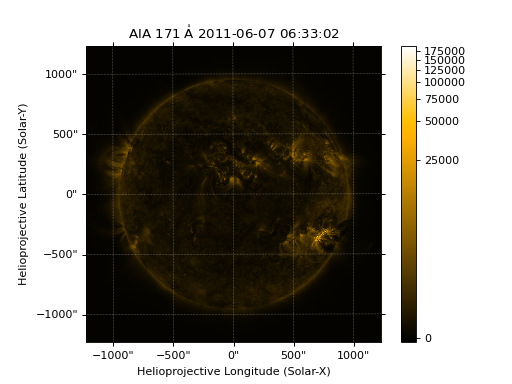### Colormaps and Normalization¶

Image data is generally shown in false color in order to better identify it or to better visualize structures in the image. Matplotlib handles this colormapping process through the colors module. This process involves two steps: the data array is first mapped onto the range 0-1 using an instance of Normalize or a subclass; then this number is mapped to a color using an instance of a subclass of a colormap.

SunPy provides the colormaps for each mission as defined by the mission teams. The Map object chooses the appropriate colormap for you when it is created as long as it recognizes the instrument. To see what colormaps are available:

>>> import sunpy.cm
>>> sunpy.cm.cmlist.keys()
dict_keys(['sdoaia94', 'sdoaia131', 'sdoaia171', 'sdoaia193', 'sdoaia211', 'sdoaia304', 'sdoaia335', 'sdoaia1600', 'sdoaia1700', 'sdoaia4500', 'sohoeit171', 'sohoeit195', 'sohoeit284', 'sohoeit304', 'soholasco2', 'soholasco3', 'stereocor1', 'stereocor2', 'stereohi1', 'stereohi2', 'rhessi', 'yohkohsxtal', 'yohkohsxtwh', 'hinodexrt', 'hinodesotintensity', 'trace171', 'trace195', 'trace284', 'trace1216', 'trace1550', 'trace1600', 'trace1700', 'traceWL', 'hmimag', 'irissji1330', 'irissji1400', 'irissji1600', 'irissji2796', 'irissji2832', 'irissji5000', 'irissjiFUV', 'irissjiNUV', 'irissjiSJI_NUV'])


The SunPy colormaps are registered with matplotlib so you can grab them like you would any other colormap:

>>> import matplotlib.pyplot as plt
>>> import sunpy.cm


You need to import sunpy.cm or sunpy.map for this to work:

>>> cmap = plt.get_cmap('sdoaia171')


The following plot shows off all of the colormaps.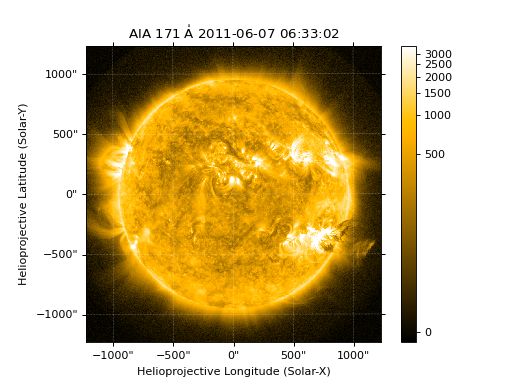These can be used with the standard commands to change the colormap. So for example if you wanted to plot an AIA image but use an EIT colormap, you would do so as follows.

import sunpy.map
import sunpy.data.sample
import matplotlib.pyplot as plt

smap = sunpy.map.Map(sunpy.data.sample.AIA_171_IMAGE)
cmap = plt.get_cmap('sohoeit171')

fig = plt.figure()
ax = plt.subplot(1,1,1)
smap.plot(cmap=cmap)
plt.colorbar()
plt.show()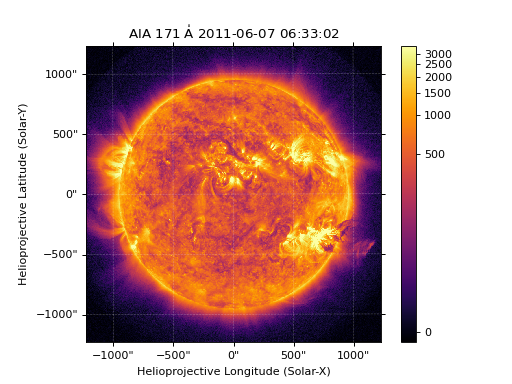or you can just change the colormap for the map itself as follows:

>>> smap.plot_settings['cmap'] = plt.get_cmap('sohoeit171')


The normalization is also set automatically and is chosen so that all the data from minimum to maximum is displayed as best as possible for most cases. This means that it is never necessary to touch the data such as applying a function such sqrt or log to the data to make your plot look good. There are many normalizations available from matplotlib such as Lognorm. Other more exotic normalizations are also made available from Astropy. Just like the colormap the default normalization can be changed through the plot_settings dictionary or directly for the individual plot by passing a keyword argument. The following example shows the difference between a linear and logarithmic normalization on an AIA image.

import sunpy.map
import sunpy.data.sample
import matplotlib.pyplot as plt
import matplotlib.colors as colors

smap = sunpy.map.Map(sunpy.data.sample.AIA_171_IMAGE)

fig = plt.figure()
ax1 = fig.add_subplot(2,1,1)
smap.plot(norm=colors.Normalize())
plt.colorbar()
ax2 = fig.add_subplot(2,1,2)
smap.plot(norm=colors.LogNorm())
fig.subplots_adjust(hspace=0.4)
plt.colorbar()
plt.show()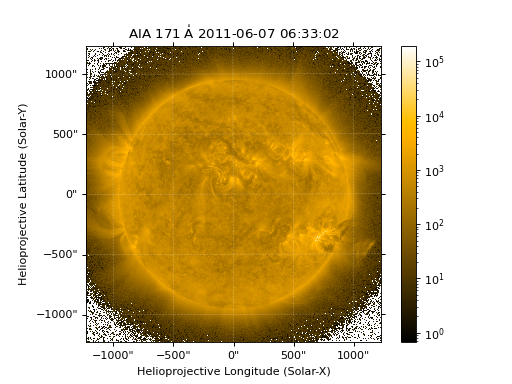Note how the color in the colorbar does not change since these two maps share the same colormap while the data values associated with each color do because the normalization is different.

## Masking and Clipping Data¶

It is often necessary for the purposes of display or otherwise to ignore certain data in an image. For example large data value could be due to cosmic ray hits and should be ignored. The most straightforward way to ignore this kind of data in plots without altering the data is to clip it. This can be achieved very easily when initializing the normalization variable. For example:

>>> import matplotlib.colors as colors
>>> norm = colors.Normalize(vmin=smap.min(), vmax=smap.mean() + 3 *smap.std())


This clips out many of the brightest pixels. If you’d like to see what areas of your images got clipped set the following values:

>>> cmap = cmap.plot_settings['cmap']
>>> cmap.set_over('red', 1.0)
>>> cmap.set_under('green', 1.0)


This will color the areas above and below in red and green respectively (similar to this example). You can use the following colorbar command to display these choices:

>>> plt.colorbar(extend='both')


Here is an example of this put to use on an AIA image. If you see how the image displays by default you’ll see that it does not look that pretty. This is because the image contains some negative values which are throwing off the normalization.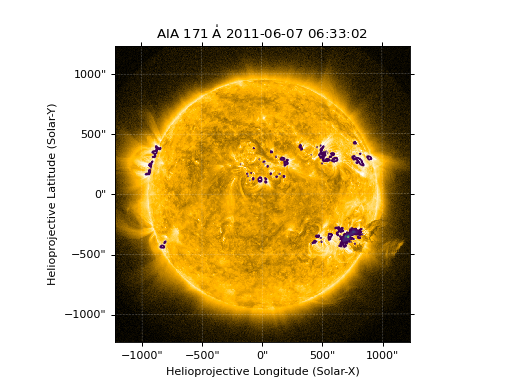In order to fix this we need to adjust our normalization to not display negative values. We can also brighten the image by clipping the high values though this will mean that the bright regions look ‘saturated’. This is achieved in the following plot.

import sunpy.map
import matplotlib.pyplot as plt
import matplotlib.colors as colors
import sunpy.data.sample
smap = sunpy.map.Map(sunpy.data.sample.AIA_193_CUTOUT01_IMAGE)
cmap = smap.plot_settings['cmap']
cmap.set_over('blue', 1.0)
cmap.set_under('purple', 1.0)
norm = colors.Normalize(vmin=0, vmax=smap.mean() + 5 * smap.std())
smap.plot(norm=norm)
plt.colorbar(extend='both')
plt.show()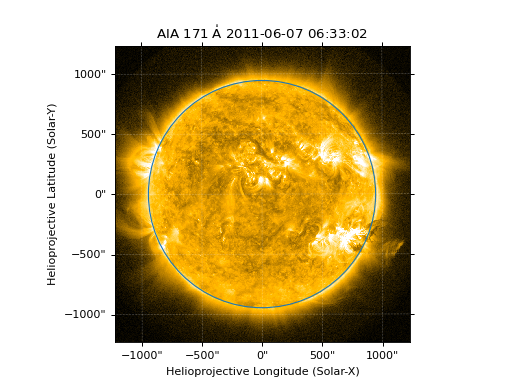Another method to ignore bad data is to mask the data. A mask is a boolean array and so can give you much more fine-grained control over what is not being displayed. A MaskedArray is a subclass of a numpy array so it has all of the same properties with the addition of an associated boolean array which holds the mask.

## Composite Maps and Overlaying Maps¶

The Map method described above can also handle a list of maps. If a series of maps are supplied as inputs, Map will return a list of maps as the output. However, if the ‘composite’ keyword is set to True, then a CompositeMap object is returned. This is useful if the maps are of a different type (e.g. different instruments). For example, to create a simple composite map:

>>> my_maps = sunpy.map.Map(sunpy.data.sample.EIT_195_IMAGE, sunpy.data.sample.RHESSI_IMAGE, composite=True)


A CompositeMap is different from a regular SunPy GenericMap object and therefore different associated methods. To list which maps are part of your composite map use:

>>> my_maps.list_maps()
[<class 'sunpy.map.sources.soho.EITMap'>, <class 'sunpy.map.sources.rhessi.RHESSIMap'>]


The following code adds a new map (which must be instantiated first), sets its transparency to 25%, turns on contours from 50% to 90% for the second map, and then plots the result.

import sunpy.data.sample
import sunpy.map
import matplotlib.pyplot as plt
my_maps = sunpy.map.Map(sunpy.data.sample.EIT_195_IMAGE, sunpy.data.sample.RHESSI_IMAGE, composite=True)
my_maps.add_map(sunpy.map.Map(sunpy.data.sample.AIA_171_IMAGE))
my_maps.set_alpha(2, 0.5)
my_maps.set_levels(1, [50, 60, 70, 80, 90], percent = True)
my_maps.plot()
plt.show()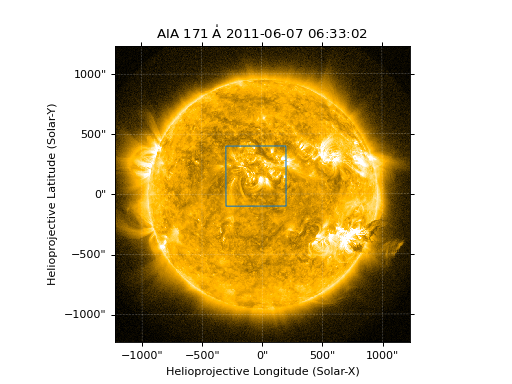This is not a particularly pretty plot but it shows what SunPy can do!

## Working with your map¶

Part of the philosophy of the map object is to provide most of the basic functionality that a scientist would want therefore a map also contains a number of map-specific methods such as resizing a map or grabbing a subview. To get a list of the methods available for a map type:

>>> help(my_map)


and check out the methods section!

## Mapcubes¶

A MapCube is an ordered list of maps. By default, the maps are ordered by their observation date, from earlier maps to later maps. A MapCube can be created by supplying multiple existing maps:

>>> map1 = sunpy.map.Map(sunpy.data.sample.AIA_171_IMAGE)
>>> map2 = sunpy.map.Map(sunpy.data.sample.EIT_195_IMAGE)
>>> mc = sunpy.map.Map([map1, map2], cube=True)


or by providing a directory full of image files:

>>> mc = sunpy.map.Map('path/to/my/files/*.fits', cube=True)


The earliest map in the mapcube can be accessed by simply indexing the maps list:

>>> mc.maps


Mapcubes can hold maps that have different shapes. To test if all the maps in a MapCube have the same shape:

>>> mc.all_maps_same_shape()
True


It is often useful to return the image data in a MapCube as a single three dimensional Numpy ndarray:

>>> mc.as_array()


Note that an array is returned only if all the maps have the same shape. If this is not true, an error (ValueError) is returned. If all the maps have nx pixels in the x-direction, and ny pixels in the y-direction, and there are n maps in the mapcube, the ndarray array that is returned has shape (ny, nx, n). The data of the first map in the MapCube appears in the ndarray in position [:, :, 0], the data of second map in position [:, :, 1], and so on. The order of maps in the MapCube is reproduced in the returned ndarray.

The meta data from each map can be obtained using:

>>> mc.all_meta()


This returns a list of map meta objects that have the same order as the maps in the MapCube.

## Coalignment of Mapcubes¶

A typical data preparation step when dealing with time series of images is to coalign images taken at different times so that features in different images remain in the same place. A common approach to this problem is to take a representative template that contains the features you are interested in, and match that to your images. The location of the best match tells you where the template is in your image. The images are then shifted to the location of the best match. This aligns your images to the position of the features in your representative template.

SunPy provides a function to coalign the maps inside the MapCube. The implementation of this functionality requires the installation of the scikit-image library, a commonly used image processing library. To coalign a MapCube, simply import the function and apply it to your MapCube:

>>> from sunpy.image.coalignment import mapcube_coalign_by_match_template
>>> coaligned = mapcube_coalign_by_match_template(mc)


This will return a new MapCube, coaligned to a template extracted from the center of the first map in the MapCube, with the map dimensions clipped as required. The coalignment algorithm provides many more options for handling the coalignment of MapCube type:

>>> help(mapcube_coalign_by_match_template)


for a full list of options and functionality.

If you just want to calculate the shifts required to compensate for solar rotation relative to the first map in the MapCube without applying them, use:

>>> from sunpy.image.coalignment import calculate_match_template_shift
>>> shifts = calculate_match_template_shift(mc)


This is the function used to calculate the shifts in MapCube coalignment function above. Please see calculate_match_template_shift to learn more about its features. Shifts calculated using calculate_match_template_shift can be passed directly to the coalignment function.

## Compensating for solar rotation in Mapcubes¶

Often a set of solar image data consists of fixing the pointing of a field of view for some time and observing. Features on the Sun will rotate according to the Sun’s rotation.

A typical data preparation step when dealing with time series of these types of images is to shift the images so that features do not appear to move across the field of view. This requires taking in to account the rotation of the Sun. The Sun rotates differentially, depending on latitude, with features at the equator moving faster than features at the poles.

SunPy provides a function to shift images in MapCube following solar rotation. This function shifts an image according to the solar differential rotation calculated at the latitude of the center of the field of view. The image is not differentially rotated. This function is useful for de-rotating images when the effects of differential rotation in the MapCube can be ignored (for example, if the spatial extent of the image is small, or when the duration of the MapCube is small; deciding on what ‘small’ means depends on your application).

To apply this form of solar derotation to a MapCube, simply import the function and apply it to your MapCube:

>>> from sunpy.physics.transforms.solar_rotation import mapcube_solar_derotate
>>> derotated = mapcube_solar_derotate(mc)


For more info see mapcube_solar_derotate.

If you just want to calculate the shifts required to compensate for solar rotation relative to the first map in the MapCube without applying them, use:

>>> from sunpy.physics.transforms.solar_rotation import calculate_solar_rotate_shift
>>> shifts = calculate_solar_rotate_shift(mc)


Please consult the docstring of the mapcube_coalign_by_match_template function in order to learn about the features of this function.